Posted in Computer graphics MCA

### The Viewing Transformation Pipeline

We know that the picture is stored in the computer memory using any convenient Cartesian co-ordinate system, referred to as World Co-Ordinate System (WCS). However, when picture is displayed on the display device it is measured in Physical Device Co-Ordinate System (PDCS) corresponding to the display device. Therefore, displaying an image of a picture involves mapping the co-ordinates of the Points and lines that form the picture into the appropriate physical device co-ordinate where the image is to be displayed. This mapping of co-ordinates is achieved with the use of co-ordinate transformation known as viewing transformation.

The viewing transformation which maps picture co-ordinates in the WCS to display co-ordinates in PDCS is performed by the following transformations.

• Converting world co-ordinates to viewing co-ordinates.

• Normalizing viewing co-ordinates.

• Converting normalized viewing co-ordinates to device co-ordinates.The steps involved in viewing transformation:-

1. Construct the scene in world co-ordinate using the output primitives and attributes.
2. Obtain a particular orientation for the window by setting a two-dimensional viewing co-ordinate system in the world co-ordinate plane and define a window in the viewing co-ordinate system.
3. Use viewing co-ordinates reference frame to provide a method for setting up arbitrary orientations for rectangular windows.
4. Once the viewing reference frame is established, transform descriptions in world co-ordinates to viewing co-ordinates.
5. Define a view port in normalized co-ordinates and map the viewing co-ordinates description of the scene to normalized co-ordinates.
6. Clip all the parts of the picture which lie outside the viewport.
Posted in Computer graphics MCA

## Composite Transformation

If a transformation of the plane T1 is followed by a second plane transformation T2, then the result itself may be represented by a single transformation T which is the composition of T1 and T2 taken in that order. This is written as T = T1∙T2.

Composite transformation can be achieved by concatenation of transformation matrices to obtain a combined transformation matrix.

A combined matrix −

[T][X] = [X] [T1] [T2] [T3] [T4] …. [Tn]

Where [Ti] is any combination of

• Translation
• Scaling
• Shearing
• Rotation
• Reflection

The change in the order of transformation would lead to different results, as in general matrix multiplication is not cumulative, that is [A] . [B] ≠ [B] . [A] and the order of multiplication. The basic purpose of composing transformations is to gain efficiency by applying a single composed transformation to a point, rather than applying a series of transformation, one after another.

For example, to rotate an object about an arbitrary point (Xp, Yp), we have to carry out three steps −

• Translate point (Xp, Yp) to the origin.
• Rotate it about the origin.
• Finally, translate the center of rotation back where it belonged.
Posted in Computer graphics MCA

## Reflection

Reflection is the mirror image of original object. In other words, we can say that it is a rotation operation with 180°. In reflection transformation, the size of the object does not change.

The following figures show reflections with respect to X and Y axes, and about the origin respectively.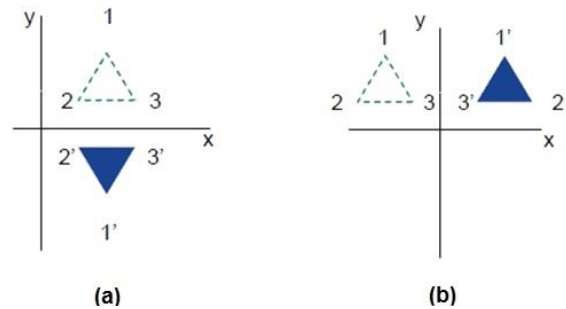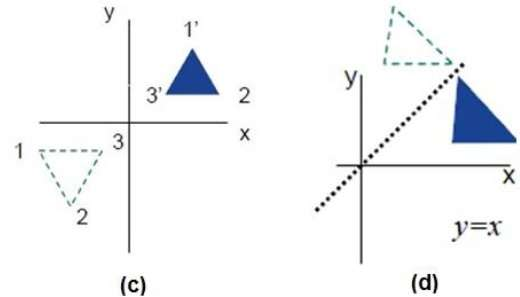## Shear

A transformation that slants the shape of an object is called the shear transformation. There are two shear transformations X-Shear and Y-Shear. One shifts X coordinates values and other shifts Y coordinate values. However; in both the cases only one coordinate changes its coordinates and other preserves its values. Shearing is also termed as Skewing.

### X-Shear

The X-Shear preserves the Y coordinate and changes are made to X coordinates, which causes the vertical lines to tilt right or left as shown in below figure.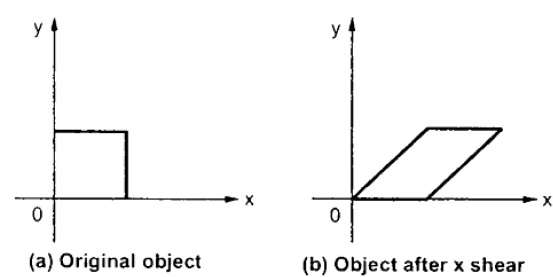The transformation matrix for X-Shear can be represented as −

Xsh=⎡⎣⎢100shx10001⎤⎦⎥Xsh=[1shx0010001]

Y’ = Y + Shy . X

X’ = X

### Y-Shear

The Y-Shear preserves the X coordinates and changes the Y coordinates which causes the horizontal lines to transform into lines which slopes up or down as shown in the following figure.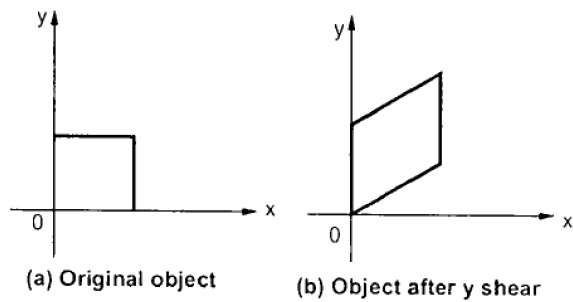The Y-Shear can be represented in matrix from as −

Ysh⎡⎣⎢1shy0010001⎤⎦⎥Ysh[100shy10001]

X’ = X + Shx . Y

Y’ = Y

Posted in Computer graphics MCA

### Matrix Representations and Homogeneous Coordinates

To perform a sequence of transformation such as translation followed by rotation and scaling, we need to follow a sequential process −

• Translate the coordinates,
• Rotate the translated coordinates, and then
• Scale the rotated coordinates to complete the composite transformation.

To shorten this process, we have to use 3×3 transformation matrix instead of 2×2 transformation matrix. To convert a 2×2 matrix to 3×3 matrix, we have to add an extra dummy coordinate W.

In this way, we can represent the point by 3 numbers instead of 2 numbers, which is called Homogeneous Coordinate system. In this system, we can represent all the transformation equations in matrix multiplication. Any Cartesian point P(X, Y) can be converted to homogeneous coordinates by P’ (Xh, Yh, h).

## Translation

A translation moves an object to a different position on the screen. You can translate a point in 2D by adding translation coordinate (tx, ty) to the original coordinate (X, Y) to get the new coordinate (X’, Y’).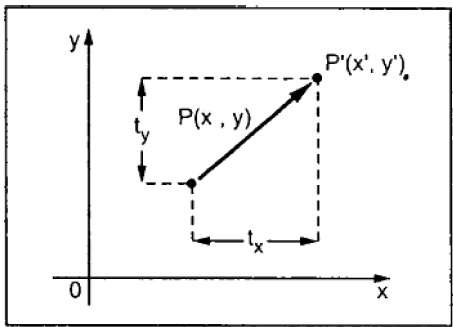From the above figure, you can write that −

X’ = X + tx

Y’ = Y + ty

The pair (tx, ty) is called the translation vector or shift vector. The above equations can also be represented using the column vectors.

P=[X][Y]P=[X][Y] p’ = [X][Y][X′][Y′]T = [tx][ty][tx][ty]

We can write it as −

P’ = P + T

## Rotation

In rotation, we rotate the object at particular angle θ (theta) from its origin. From the following figure, we can see that the point P(X, Y) is located at angle φ from the horizontal X coordinate with distance r from the origin.

Let us suppose you want to rotate it at the angle θ. After rotating it to a new location, you will get a new point P’ (X’, Y’).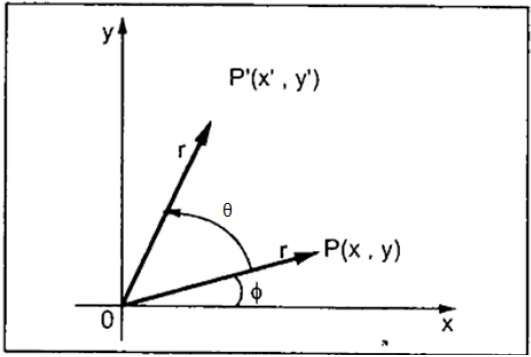Using standard trigonometric the original coordinate of point P(X, Y) can be represented as −

X=rcosϕ......(1)X=rcosϕ……(1)

Y=rsinϕ......(2)Y=rsinϕ……(2)

Same way we can represent the point P’ (X’, Y’) as −

x=rcos(ϕ+θ)=rcosϕcosθrsinϕsinθ.......(3)x′=rcos(ϕ+θ)=rcosϕcosθ−rsinϕsinθ…….(3)

y=rsin(ϕ+θ)=rcosϕsinθ+rsinϕcosθ.......(4)y′=rsin(ϕ+θ)=rcosϕsinθ+rsinϕcosθ…….(4)

Substituting equation (1) & (2) in (3) & (4) respectively, we will get

x=xcosθysinθx′=xcosθ−ysinθ

y=xsinθ+ycosθy′=xsinθ+ycosθ

Representing the above equation in matrix form,

[XY]=[XY][cosθsinθsinθcosθ]OR[X′Y′]=[XY][cosθsinθ−sinθcosθ]OR

P’ = P . R

Where R is the rotation matrix

R=[cosθsinθsinθcosθ]R=[cosθsinθ−sinθcosθ]

The rotation angle can be positive and negative.

For positive rotation angle, we can use the above rotation matrix. However, for negative angle rotation, the matrix will change as shown below −

R=[cos(θ)sin(θ)sin(θ)cos(θ)]R=[cos(−θ)sin(−θ)−sin(−θ)cos(−θ)]
=[cosθsinθsinθcosθ](cos(θ)=cosθandsin(θ)=sinθ)=[cosθ−sinθsinθcosθ](∵cos(−θ)=cosθandsin(−θ)=−sinθ)

## Scaling

To change the size of an object, scaling transformation is used. In the scaling process, you either expand or compress the dimensions of the object. Scaling can be achieved by multiplying the original coordinates of the object with the scaling factor to get the desired result.

Let us assume that the original coordinates are (X, Y), the scaling factors are (SX, SY), and the produced coordinates are (X’, Y’). This can be mathematically represented as shown below −

X’ = X . SX and Y’ = Y . SY

The scaling factor SX, SY scales the object in X and Y direction respectively. The above equations can also be represented in matrix form as below −

(XY)=(XY)[Sx00Sy](X′Y′)=(XY)[Sx00Sy]

OR

P’ = P . S

Where S is the scaling matrix. The scaling process is shown in the following figure.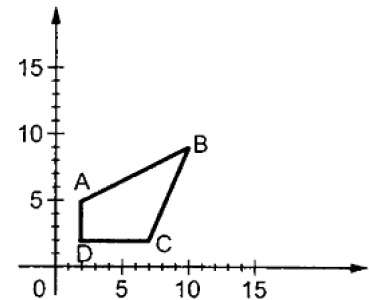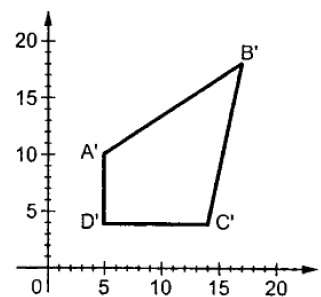If we provide values less than 1 to the scaling factor S, then we can reduce the size of the object. If we provide values greater than 1, then we can increase the size of the object.

Posted in Computer graphics MCA

### Two-dimensional Geometric Transformations

2-D Transformation is a basic concept in computer graphics. We’ll cover it in brief as there are many important aspects to it that need to be discussed. So, what do we mean by 2-D transformations?

In the context of computer graphics, it means to alter the orientation, size, and shape of an object with geometric transformation in a

2-D plane. Now, a question arises: What geometric transformations?

Well, we use three basic transformations: Translation, Rotation, and Scaling. Let’s learn about each of them.

1) TRANSLATION: Unlike Rotation, the word ‘translation’ don’t click at first, it’s because we don’t use this word in our day to day lives. But, it’s a very simple and yet a powerful concept. The definition says, repositioning an object along a straight line path from one coordinate location to another. Or in simple words to move an object in 2-D space we can use translation. There is an important point to note here, we are not changing the size or orientation of the object in any way, i.e. we don’t resize or rotate the object, we just move it to some other coordinates.

In order to move an object in 2-D space, we need to add/subtract some value from its x and y coordinates. That distance is known as

translational distance‘. One more thing to remember here is that the translational distance pair (tx, ty) is called translation vector or shift vector. New positions (x’y’) in terms of old coordinate positions (xy) can be described as:

x’ = x + tx,    y’ = y + t

We can express the translational equation above as a single matrix equation by using column vectors to represent coordinate positions and translational vectors.

P=[x1x2] , P’= [x1x2],  T= [txty]

Thus, 2-D translation equation in matrix form can be written as P’ =P +T

2) ROTATION:  We use this word very frequently in day to day life. In computer graphics, it means the same. To reposition an object along a circular path in the xy plane is called Rotation. There are two things needed for rotation, θ the rotation angle and the position (x, yr) of the rotation point or pivot point.

Note: Positive values for rotation angle define counter-clockwise direction about the pivot point and vice versa.

With column vector representation, P= R.P

where R= [cosθsinθsinθcosθ]

3) SCALING: In simple words, scaling transformation alters the size of an object. It can be achieved by multiplying the coordinate values (x, y) of each vertex by multiplying by scaling factors (sx , sy) .

x’= x.sx,  y’= y.sy

With column vector representation, P’ = S.P

where S (scaling matrix)= [sx00sy]

Also, we can do scaling in two ways: 1) Uniform Scaling 2) Differential Scaling

1) Uniform Scaling: When sand sy are assigned the same values

2) Differential Scaling: Unequal values of sx and sy result in a differential scaling

Posted in Computer graphics MCA

### Anti-aliasing in computer graphics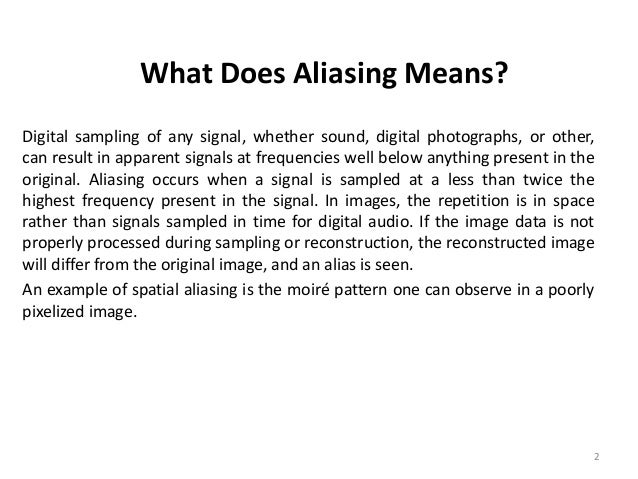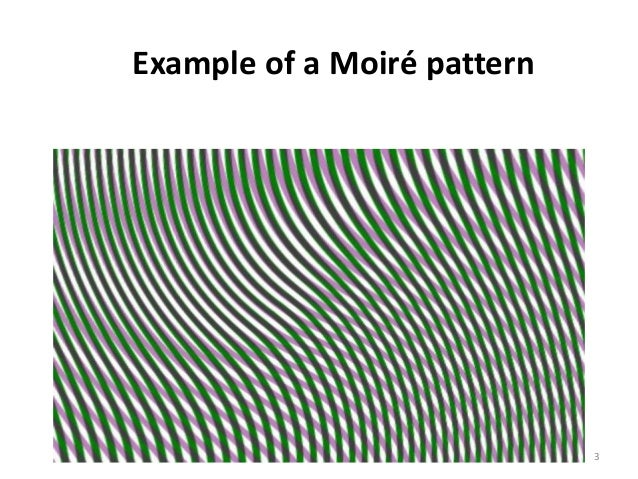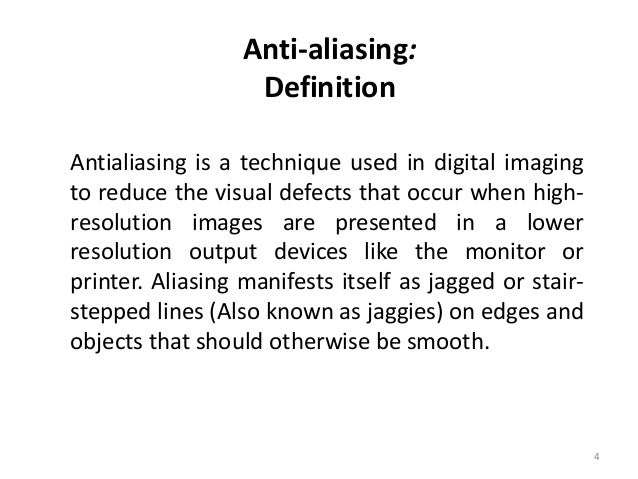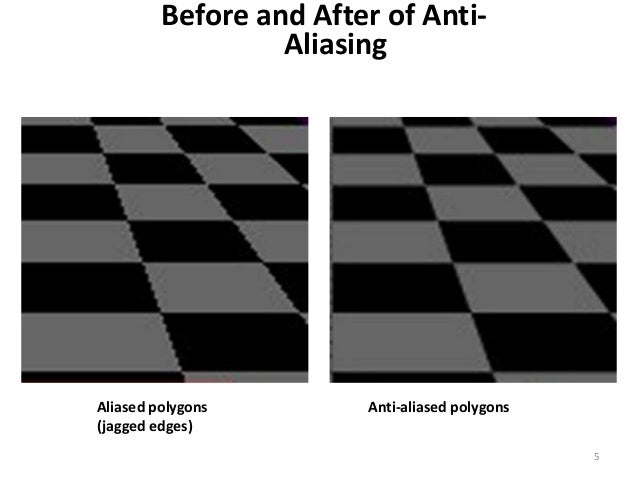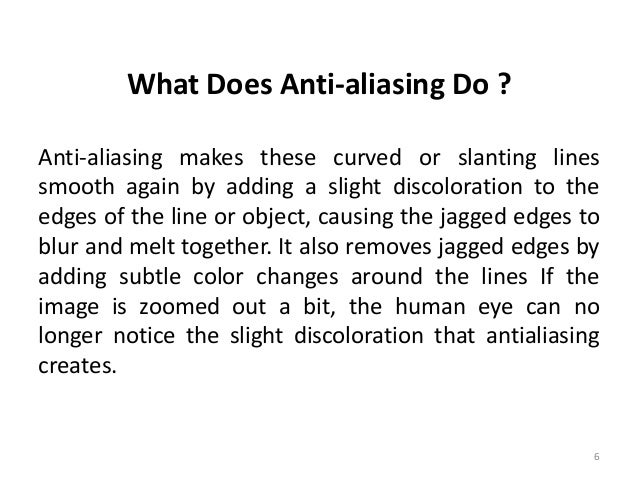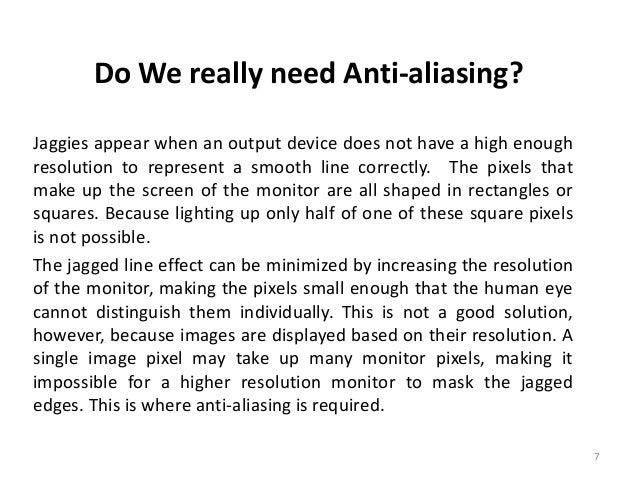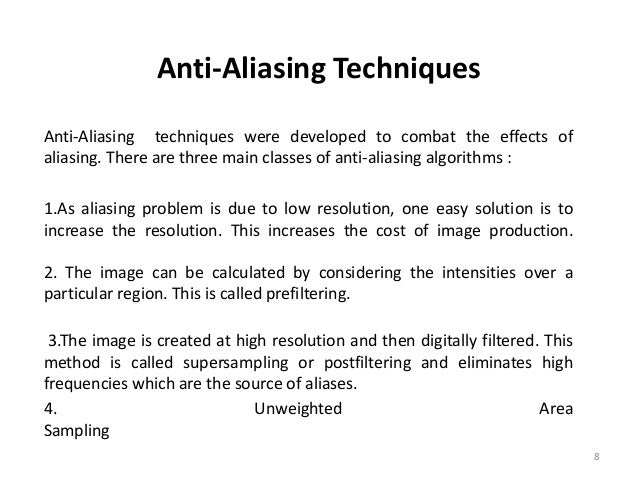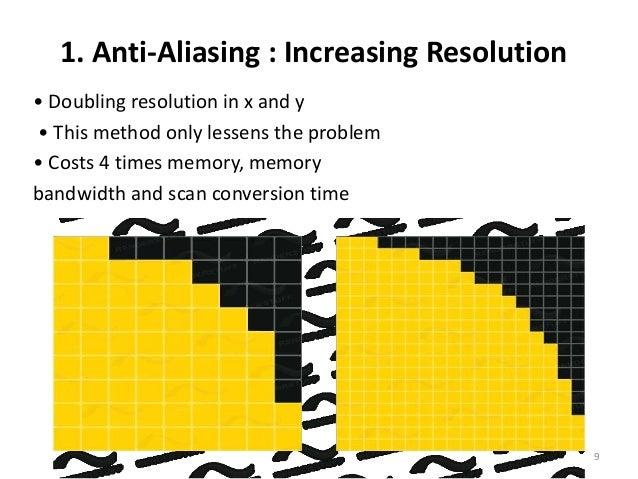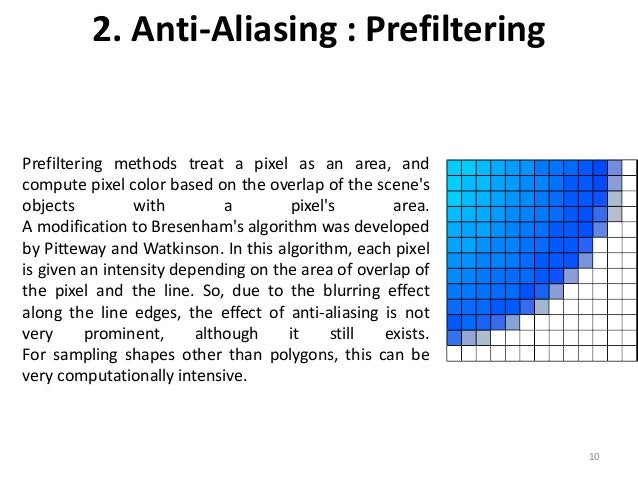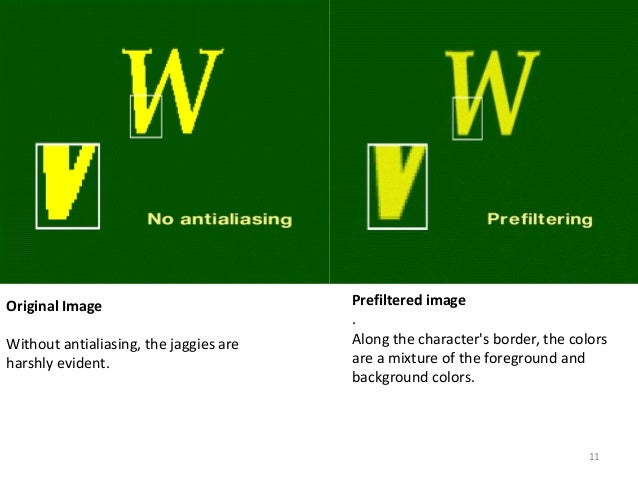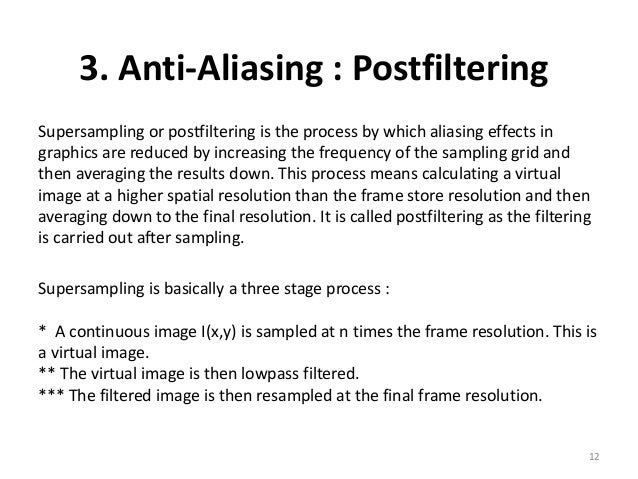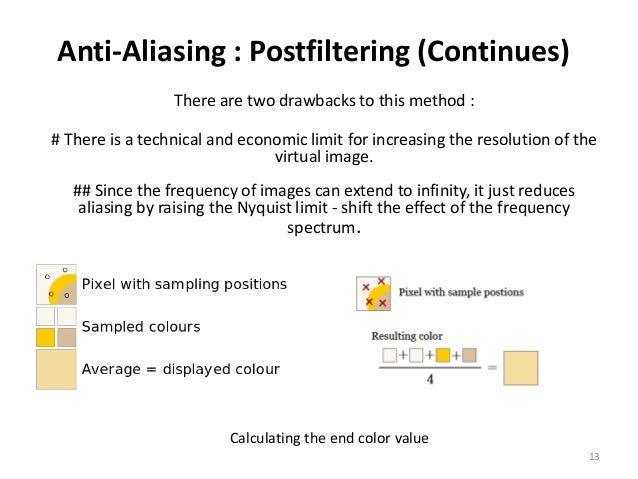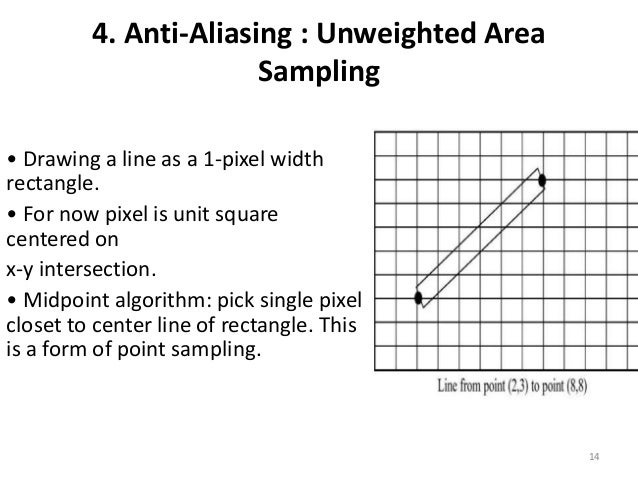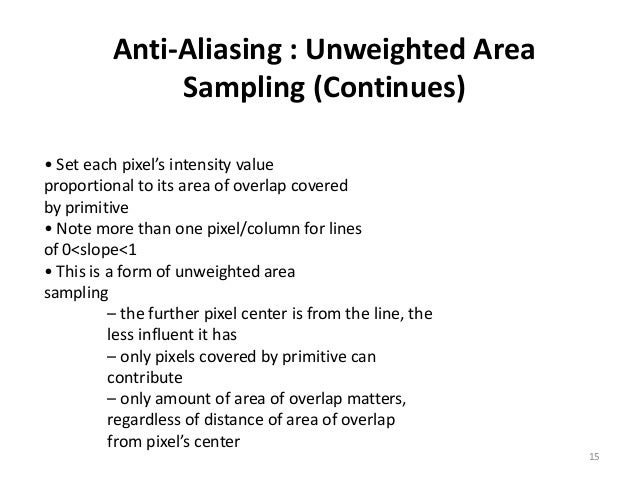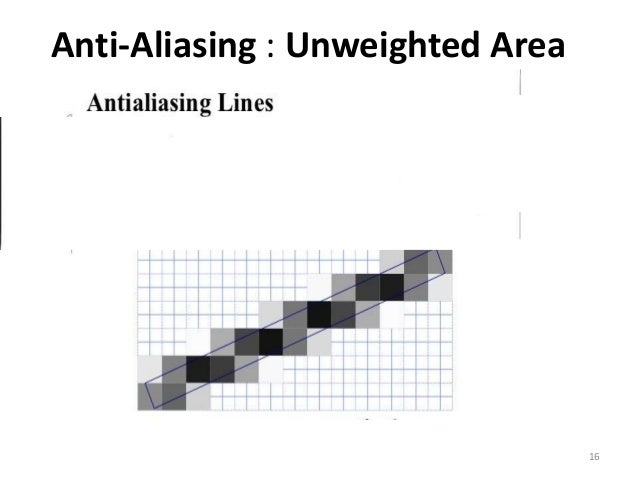### Anti-Aliasing

• Raster algorithms generate jagged edges
• Discrete sampling of continuous function
• Undersampling (low frequency sampling) causes aliasing

Nyquest sampling frequency ( f)

f= 2 fmax

n = c / l = (nm/sec)/(nm/cycle) = cycles/sec

c = nm / sec = speed of light

n (wavelength/second)

l = nm / wavelength

cycle = wavelength

and

Dxsample Dxcycles / 2

Nyquest sampling interval for Dxsample  interval

where Dxcycles = 1 / fmax     or       fmax =  1 / Dxcycles

as above          n a 1 / l

• Raster graphics increases resolution but
• Frame buffer has limits
• Require arbitrary resolution

Super sampling => for images

• increase sampling rate by treating screen as if covered with finer grid
• use multiple sampling points across finer grid to determine appropriate intensity level for each pixel

Lines =>

• Calculate area of overlap for each pixel => area sampling

## Super sampling straight lines

Solutions

1. For greyscale display of straight line:
• Divide each pixel into subpixels
• Count number of pixels along line path
• Set pixel intensity to subpixel count

o      e.g. divide each pixel in the 3 x 3 = 9 subpixels

o      use Bresenham’s algorithm to determine coverage

o      Four intensity levels – including black

• all 3pixels on line
• 2 pixels on line
• 1 pixel on line
• 0 pixel on line
1. Finite size line width

Super sample

• Set each pixel intensity proportional to number of pixels inside polygon representing line area
• Subpixel in line if LLC of pixel is inside polygon boundary

o      Adv. => number of available intensities = total number of pixels in area

o      Adv. => total line intensity distributed over more pixels

• e.g. 5 pixels in red, 4 in blue
• Pixel color = (5 * red + 4 * blue)/9

• Super sampling gives weight to interior pixels

e.g.

 2 1 2 1 1 2 4 2 0 1 2 1 0 1 2

Sum of matrix element values = 16

Weight = value / sum

e.g.    at (1,1) weight = 4 / 16/ = ¼

at (0,0) weight = 1 /16

Posted in Computer graphics MCA

### Bundled Attributes in computer graphics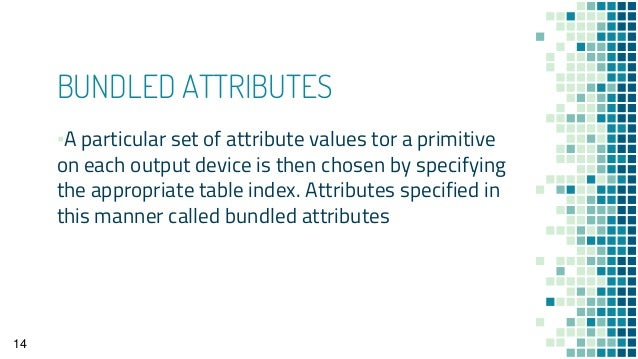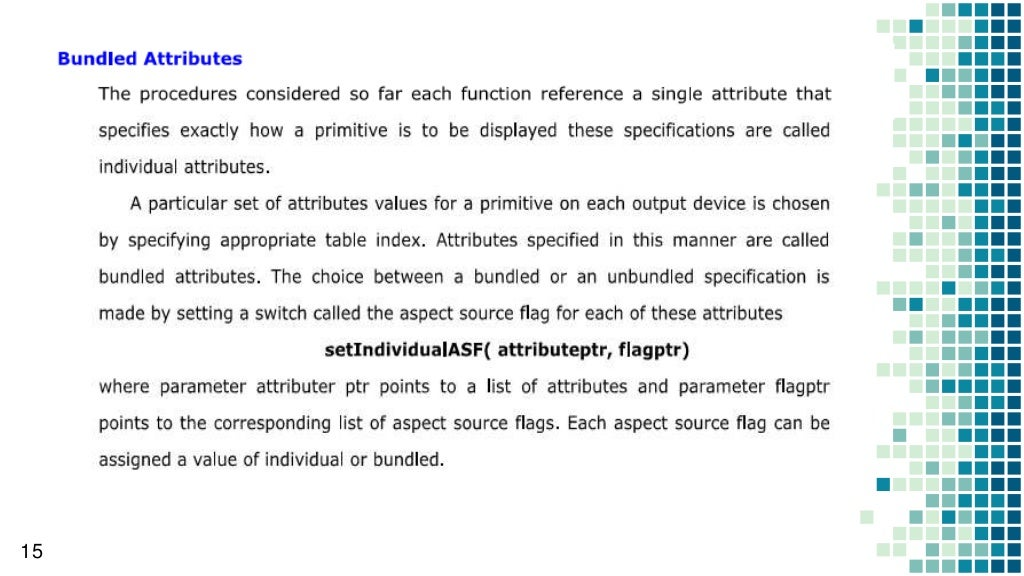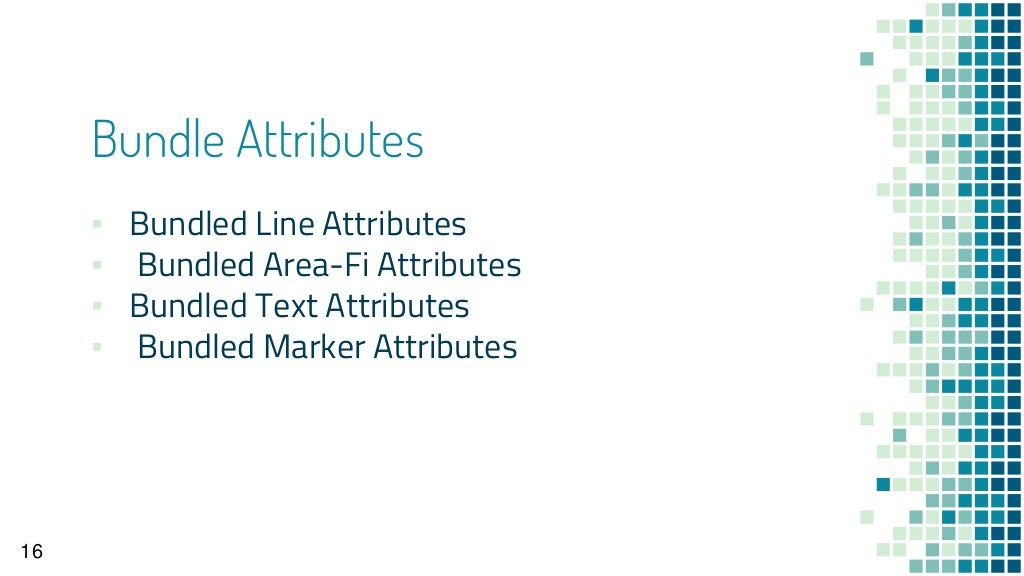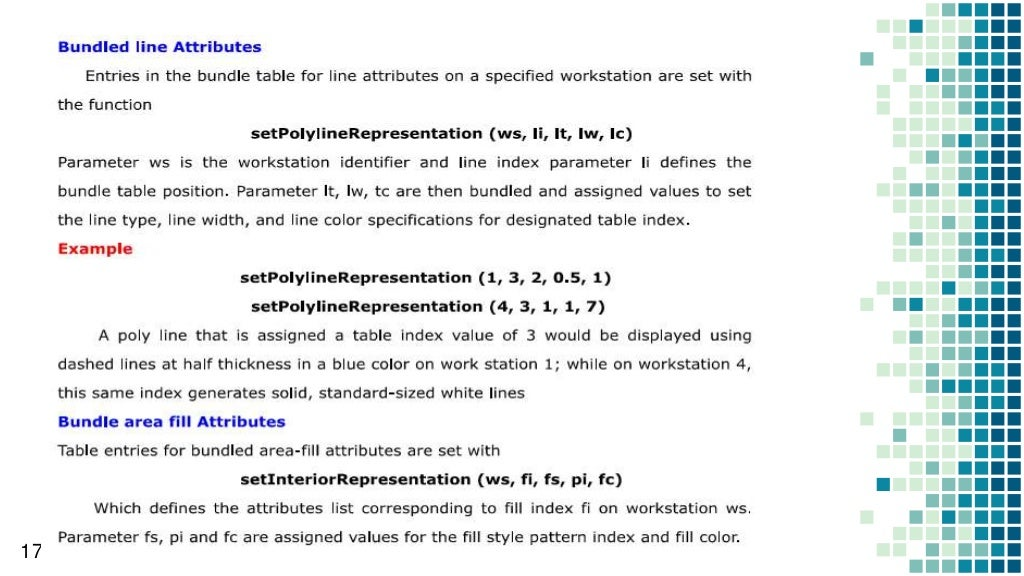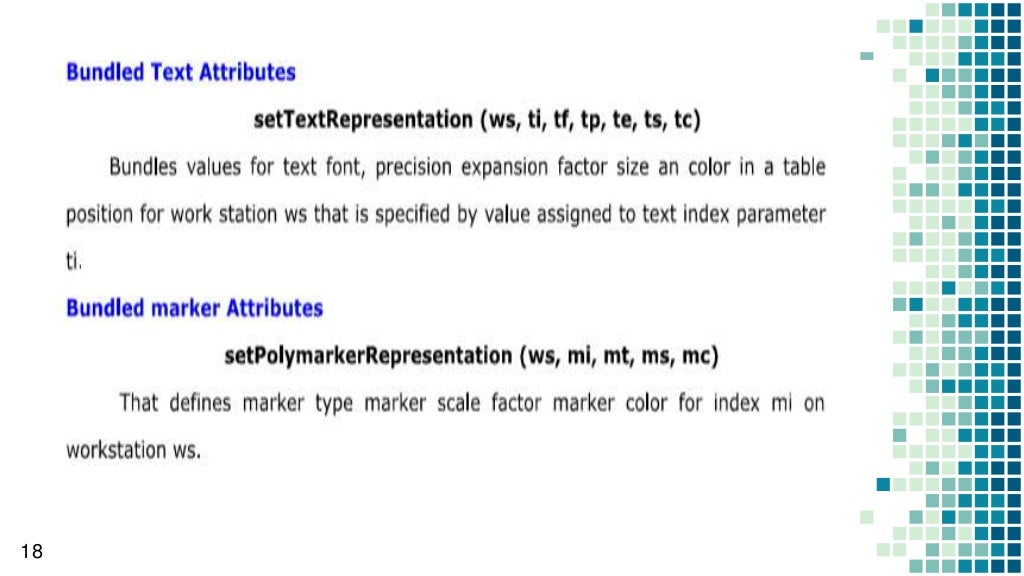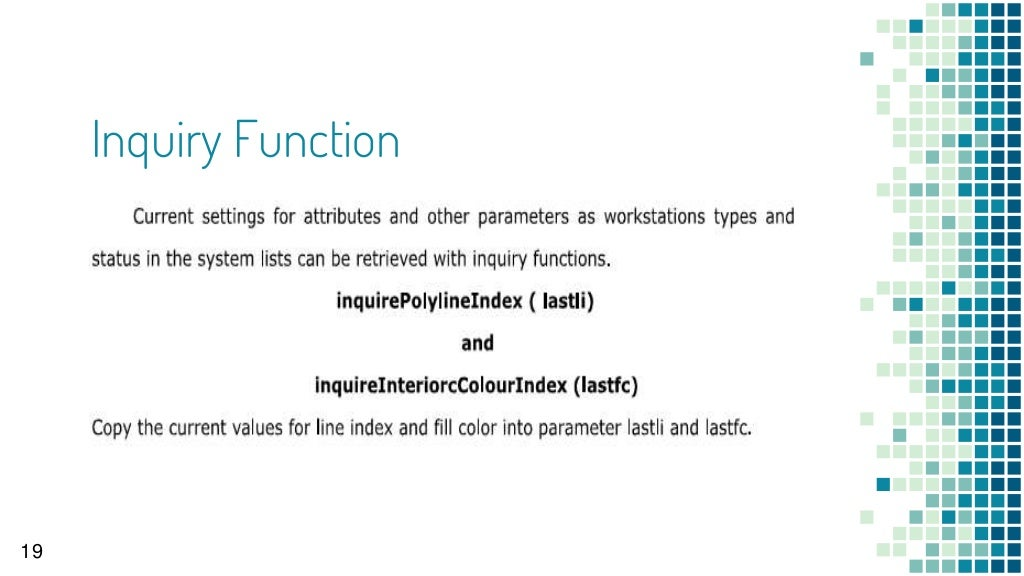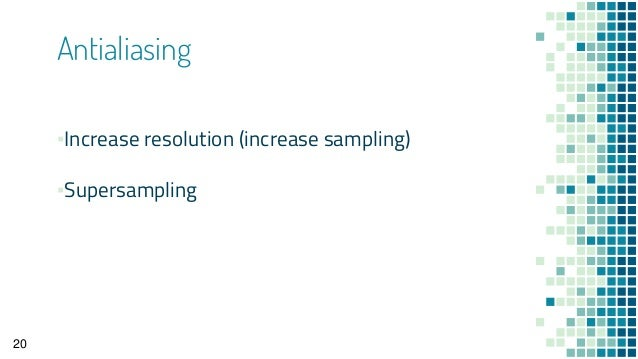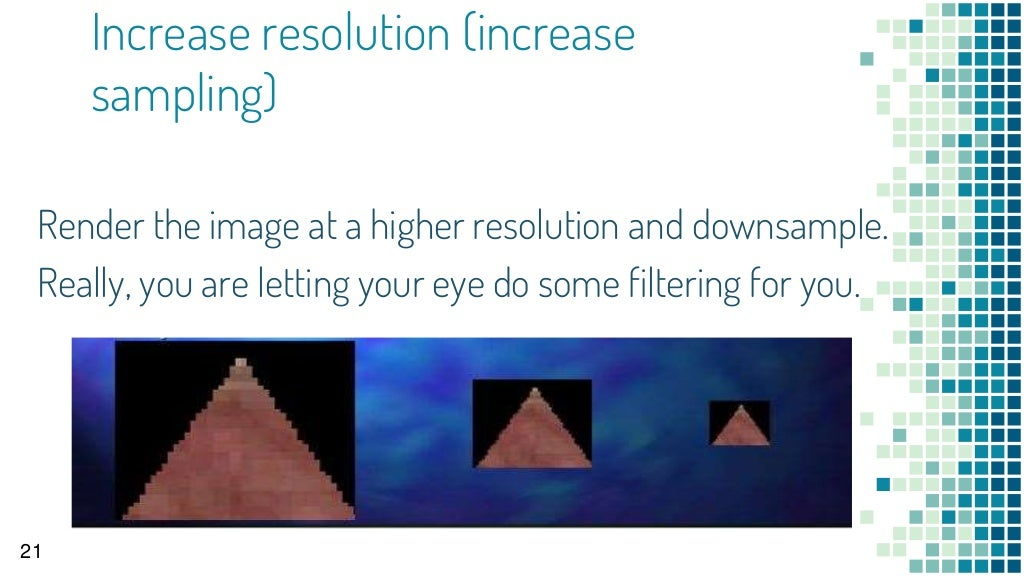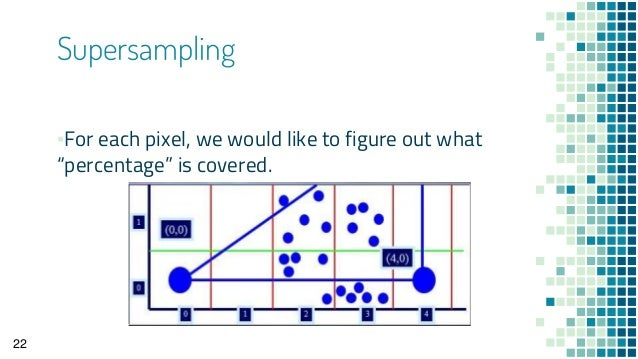Posted in Computer graphics MCA

### Character Attributes in computer graphics

Attributes – font, size, color, orientation

Text Attributes

Assorted underlying styles (solid, double, dotted)

Scale height and width

Size specified in points

1 pt = .013837 inches or 1/72 inch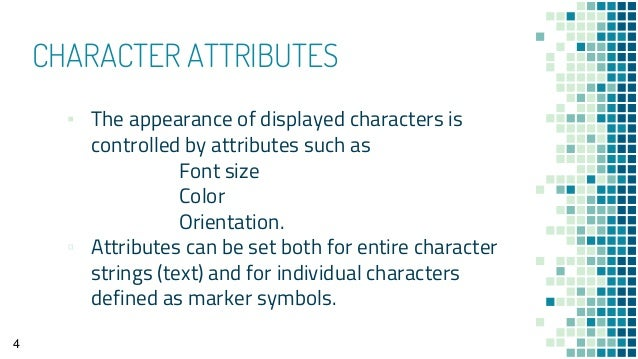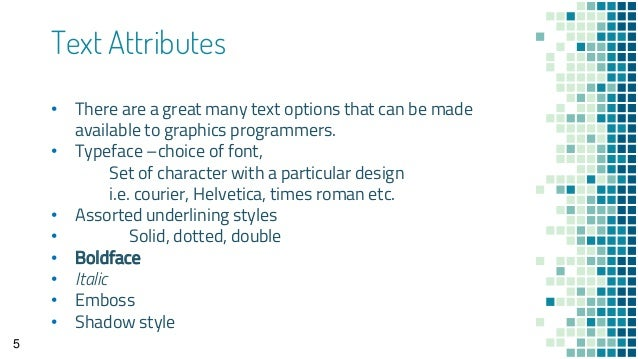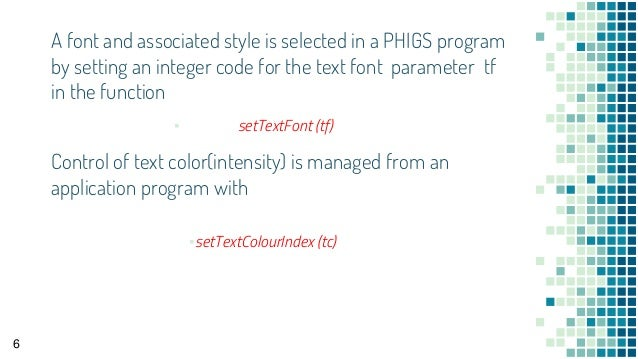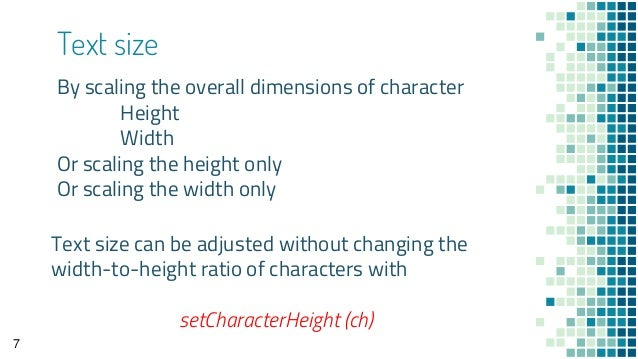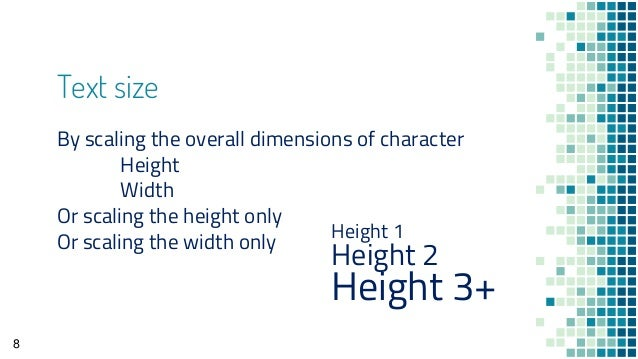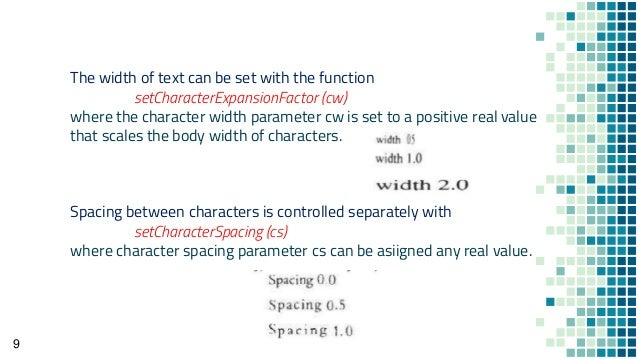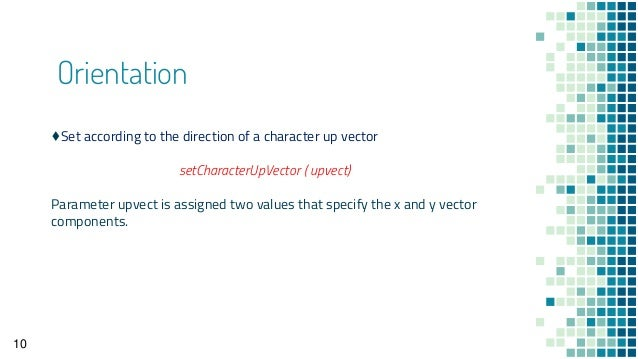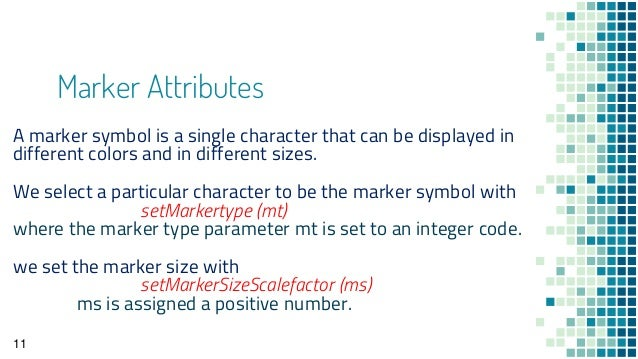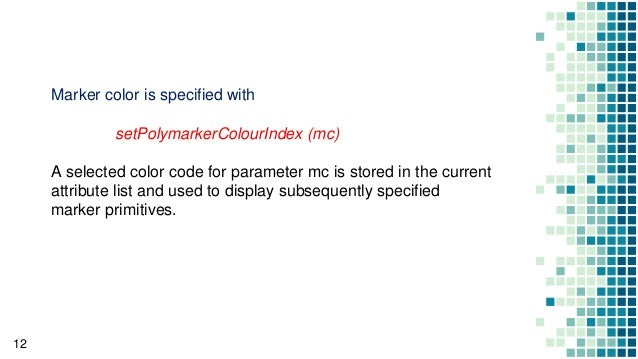Posted in Computer graphics MCA

### Color and Grayscale Levels

Grayscale is a range of shades of gray without apparent color. The darkest possible shade is black, which is the total absence of transmitted or reflected light. The lightest possible shade is white, the total transmission or reflection of light at all visible wavelength s. Intermediate shades of gray are represented by equal brightness levels of the three primary colors (red, green and blue) for transmitted light, or equal amounts of the three primary pigments (cyan, magenta and yellow) for reflected light.

In the case of reflected light (for example, in a printed image), the levels of cyan (C), magenta (M), and yellow (Y) for each pixel are represented as a percentage from 0 to 100. For each pixel in a cyan-magenta-yellow (CMY) grayscale image, all three primary pigments are present in equal amounts. That is, C = M = Y. The lightness of the gray is inversely proportional to the number representing the amounts of each pigment. White is thus represented by C = M = Y = 0, and black is represented by C = M = Y = 100.

In some systems that use the RGB color model, there are 2 16 , or 65,636, possible levels for each primary color. When R = G = B in this system, the image is known as 16-bit grayscale because the decimal number 65,536 is equivalent to the 16-digit binary number 1111111111111111. As with 8-bit grayscale, the lightness of the gray is directly proportional to the number representing the brightness levels of the primary colors. As one might expect, a 16-bit digital grayscale image consumes far more memory or storage than the same image, with the same physical dimensions, rendered in 8-bit digital grayscale.

In analog practice, grayscale imaging is sometimes called “black and white,” but technically this is a misnomer. In true black and white, also known as halftone, the only possible shades are pure black and pure white. The illusion of gray shading in a halftone image is obtained by rendering the image as a grid of black dots on a white background (or vice-versa), with the sizes of the individual dots determining the apparent lightness of the gray in their vicinity. The halftone technique is commonly used for printing photographs in newspapers.

In some cases, rather than using the RGB or CMY color models to define grayscale, three other parameters are defined. These are hue, saturation and brightness . In a grayscale image, the hue (apparent color shade) and saturation (apparent color intensity) of each pixel is equal to 0. The lightness (apparent brightness) is the only parameter of a pixel that can vary. Lightness can range from a minimum of 0 (black) to 100 (white).

Posted in Computer graphics MCA

### CHARACTER GENERATION IN COMPUTER GRAPHICS

Computer graphics involves display of picture, lines and other graphics like designs.These picture and graph will belongs to some data. Some information and instruction should be given to the user about this data. This is posssible with the help of text display.

Since text consists of string of characters. so a character is a basc unit of text
There are three methods for character generation. These are:
1) Stroke Method
2) Bitmap Method
3) Starbust Method

##### 1)STROKE METHOD

Stroke method is based on natural method of text written by human being. In this method graph is drawing in the form of line by line.
Line drowing algorithm DDA follow this method for line drawing.

##### 2)BITMAP METHOD

Bitmap method is a called dotmatrix method . as the name suggest this method use array of bits fot generating a character. This dots are the points for array whose size is fixed.
In bitmatrix method when the dots is stored in the form of array the value 1 in array represent the characters i.e where the dots appear we represent that position with numerical value 1 and the value where dots are not present is reprented by 0 in array.

##### 3)STARBUST METHOD

Starbust method is user in a particular pattern where only 24 strokes are defined for character generation

### This program is for Character Generation.

#include<stdio.h>

#include<conio.h>

#include<graphics.h>

main()

{

int gd,gm,i,j;

/* Save character map of letter A */

// You can make your changes in the below array

int a = {

{ 0, 0, 0, 0, 1, 0, 0, 0, 0},

{ 0, 0, 0, 1, 0, 1, 0, 0, 0},

{ 0, 0, 1, 0, 0, 0, 1, 0, 0},

{ 0, 1, 0, 0, 0, 0, 0, 1, 0},

{ 0, 1, 0, 0, 0, 0, 0, 1, 0},

{ 0, 1, 0, 0, 0, 0, 0, 1, 0},

{ 0, 1, 1, 1, 1, 1, 1, 1, 0},

{ 0, 1, 0, 0, 0, 0, 0, 1, 0},

{ 0, 1, 0, 0, 0, 0, 0, 1, 0},

{ 0, 1, 0, 0, 0, 0, 0, 1, 0},

{ 0, 1, 0, 0, 0, 0, 0, 1, 0},

{ 0, 1, 0, 0, 0, 0, 0, 1, 0},

{ 0, 1, 0, 0, 0, 0, 0, 1, 0},

};

/* Initialise graphics mode */

detectgraph(&gd,&gm);

initgraph(&gd,&gm,”c:\\tc\\bgi”);

for(i=0;i<13;i++)

{

for(j=0;j<9;j++)

{

putpixel(200+j,200+i,15*a[i][j]);

}

}

getch();

closegraph();

}